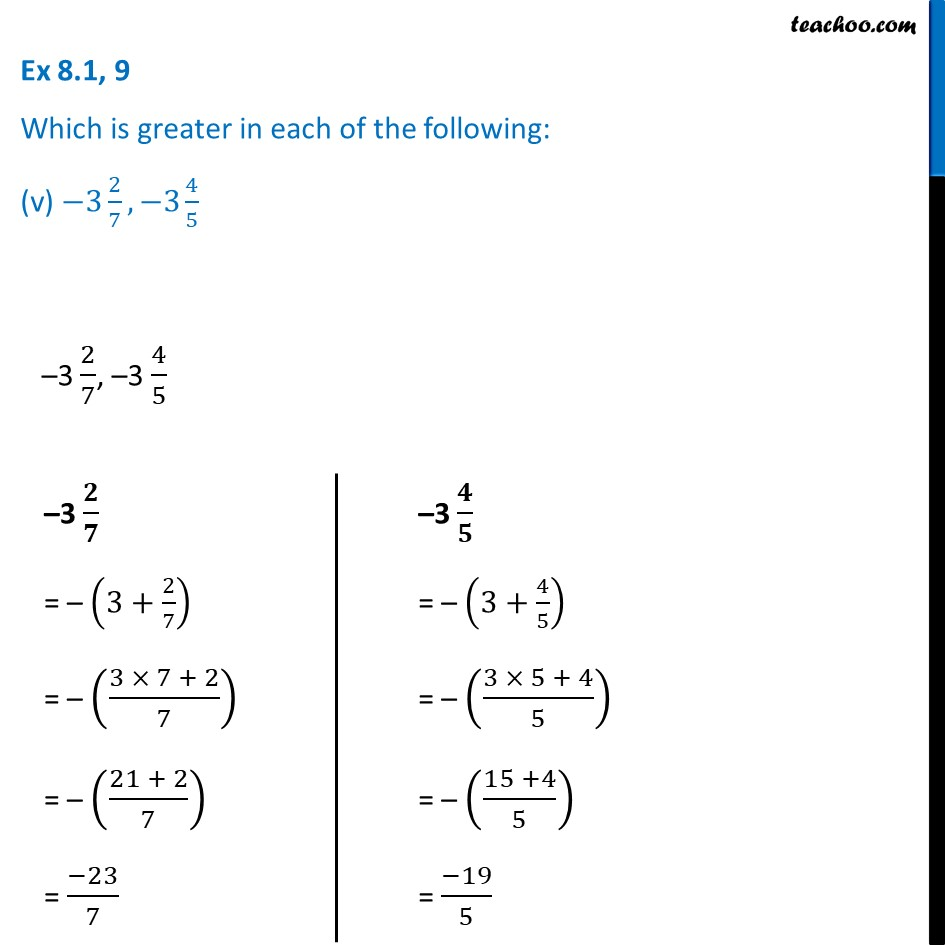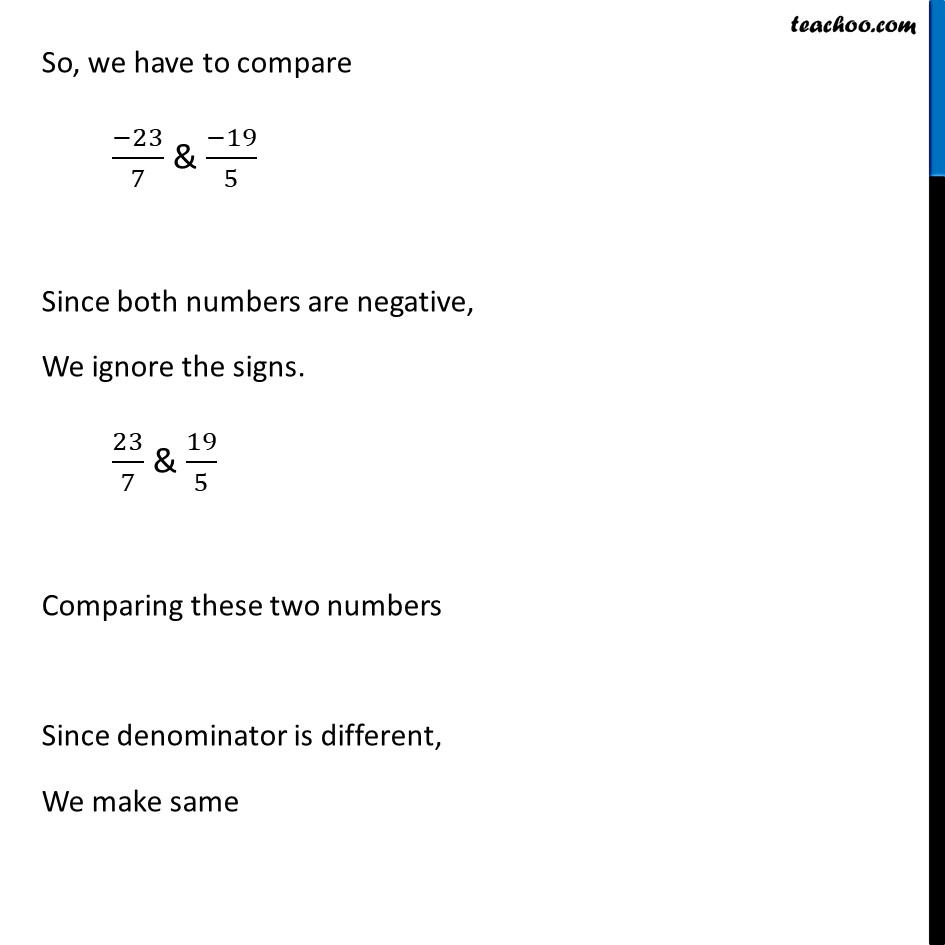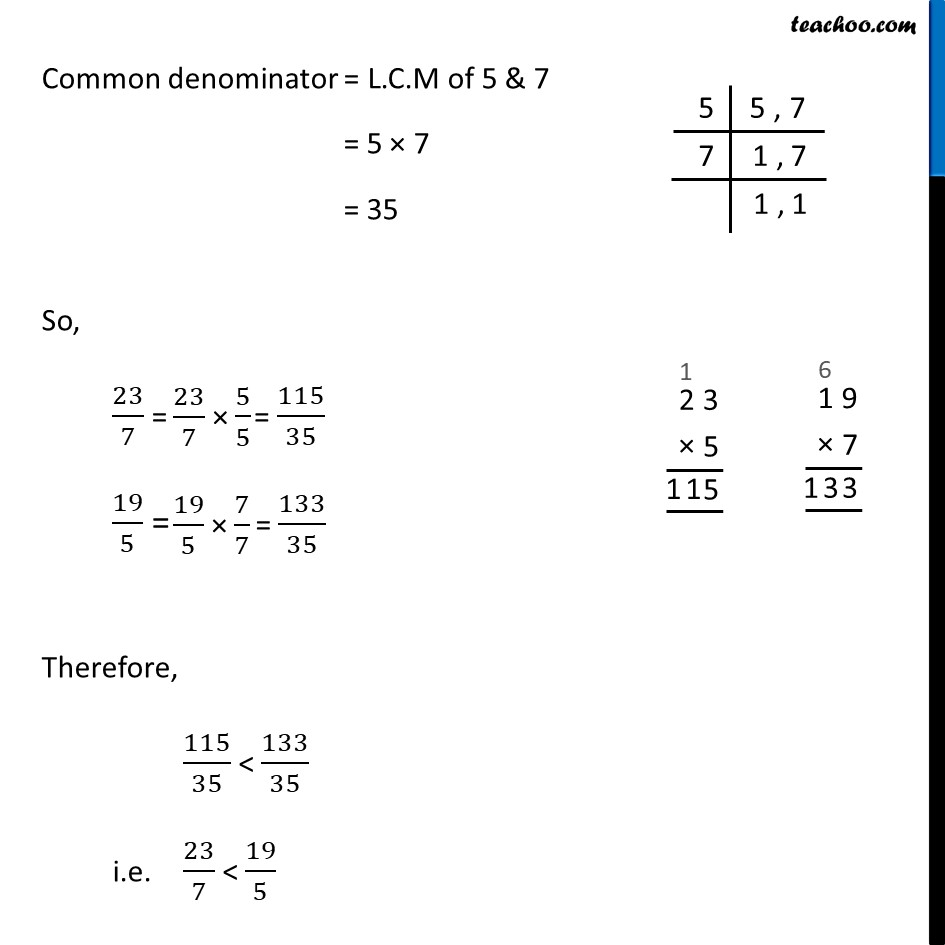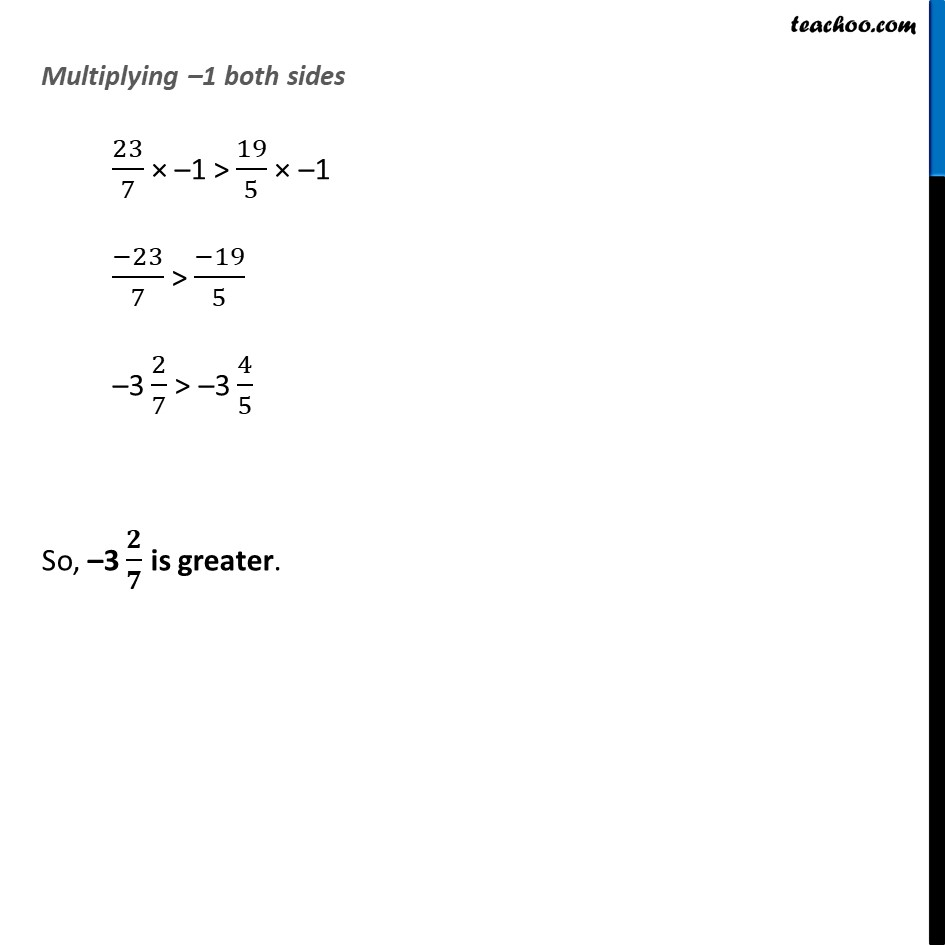Ex 8.1

Chapter 8 Class 7 Rational Numbers
Serial order wiseLearn in your speed, with individual attention - Teachoo Maths 1-on-1 Class

### Transcript

Ex 8.1, 9 Which is greater in each of the following: (v) −3 2/7, −3 4/5 –3 𝟐/𝟕 = – (3+2/7) = – ((3 × 7 + 2)/7) = – ((21 + 2)/7) = (−23)/7 –3 𝟒/𝟓 = – (3+4/5) = – ((3 × 5 + 4)/5) = – ((15 +4)/5) = (−19)/5 So, we have to compare (−23)/7 & (−19)/5 Since both numbers are negative, We ignore the signs. 23/7 & 19/5 Comparing these two numbers Since denominator is different, We make same Common denominator = L.C.M of 5 & 7 = 5 × 7 = 35 So, 23/7 = 19/5 = Therefore, 115/35 < 133/35 i.e. 23/7 < 19/5 Multiplying –1 both sides 23/7 × –1 > 19/5 × –1 (−23)/7 > (−19)/5 –3 2/7 > –3 4/5 So, –3 𝟐/𝟕 is greater.